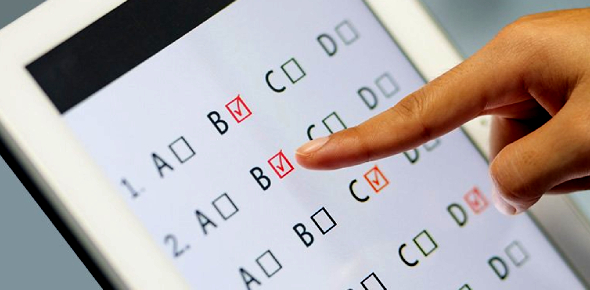20 QuestionsSettingsCreate a 20 question fixed response quiz about any of our course content that we have covered so far. 10 items should be multiple choice; 10 should be true/false. In each set, five items should be examples; five should be non-examples. You may create the quiz in an software you wish to, as long as everyone in the class has access to it.

Related Topics
• 1.
What are the four components of a performance objective?
• A.

Condition, constraints, constants, criteria

• B.

Audience, behavior, condition, degree

• C.

Audience, time allowed, location, degree

• D.

Behavior, location, time allowed, degree

• 2.
What is a characteristic of distance education?
• A.

Course management software selected

• B.

The subject matter

• C.

Number of miles between instructor and learners

• D.

Separation of instructor and learners

• 3.
Malcolm Knowles is known for which theory?
• A.

Equivalency

• B.

Andragogy

• C.

Fordism

• D.

Independent Study

• 4.
Which of the following is an example of a Web 2.0 technology?
• A.

Local area networks

• B.

Firewalls

• C.

COBOL

• D.

Podcasting

• 5.
Which of the following is not a model for designing online courses?
• A.

Linear-designed instruction

• B.

Branched-designed instruction

• C.

Learner-directed design

• D.

Hybrid-content design

• 6.
An example of an objective assessment
• A.

Is an essay test

• B.

Is a true/false question

• C.

Is an ePortfolio

• D.

Is the summary of "War and Peace"

• 7.
Select one consideration that instructors do not have to be concerned with when planning to teach an online class.
• A.

Cost of the class

• B.

Number of students in the class

• C.

Subject matter

• D.

Other classes students are taking

• 8.
Sampling error
• A.

Is when the sample set's responses are recorded incorrectly.

• B.

Is a mathematical error in computing the statistical mean.

• C.

Is the difference between everyone responding and the sample set responding.

• D.

Is sampling a grumpy group of people.

• 9.
As defined by cognitive psychologists, there are three types of capabilities that all people possess.  Select the option that is not one of these three capabilities.
• A.

Declarative knowledge

• B.

Problem-solving

• C.

Procedural knowledge

• D.

Know-how

• 10.
The second generation of distance learning technologies was from the years
• A.

1942 - 1999

• B.

2000 - 2005

• C.

1960 - 1985

• D.

1970 - 1975

• 11.
Teacher-centered learning accurately describes distance education.
• A.

True

• B.

False

• 12.
Summative assessments are designed to refine the instruction.
• A.

True

• B.

False

• 13.
A rubric is designed to guide the evaluation of a complex assignment.
• A.

True

• B.

False

• 14.
Performance objectives and learning objectives are the same.
• A.

True

• B.

False

• 15.
Validity is the degree to which a test measures what it is supposed to measure.
• A.

True

• B.

False

• 16.
The digital divide means that students must sit in separate places in face-to-face classes.
• A.

True

• B.

False

• 17.
Adult learners are always better suited to online classes than K-12 students.
• A.

True

• B.

False

• 18.
Plagiarism and cheating always mean the same thing.
• A.

True

• B.

False

• 19.
Distance education means how far the student must travel to attend a face-to-face class.
• A.

True

• B.

False

• 20.
A performance objective is designed to specify how to behave when taking a test.
• A.

True

• B.

False